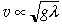Understanding Basic StatisticsExcelFittingKaleidagraphOriginPower Laws • Dimensional Analysis

# Dimensional Analysis

First used by J. B. J. Fourier in 1822, dimensional analysis is a simple and powerful technique for learning about the dependence of a phenomenon on the dimensions and properties of the system that exhibits the phenomenon. It is based on the simple principle that the units on each side of any valid physical equation must be the same.

The principle is perhaps best explains by an example. You are all familiar with the phenomenon of water waves on the surface of the ocean. What determines the speed of the waves?

To apply dimensional analysis, we must identify the properties of the system that might influence the wave speed. Let's draw up a list of possibilities:

Potentially Relevant(Probably) Irrelevant
• density of water, ρ
• acceleration of gravity, g
• wavelength, λ
• ocean depth, h
• temperature
• salinity
• density of air

You might be able to add some others to the list, but I'll work with these. We would like to find an expression for the speed v of the wave. It has dimensions of length per unit time, which I'll symbolize

[v] = L T-1        (1)

To see what combination of the relevant variables produces a quantity with the dimensions of velocity, write out the dimensions of each one:

 ρ M L-3 g L T-2 λ L h L

A couple of things stand out right away. First, the only quantity having mass in it is ρ. Since v doesn't have any M, it cannot depend on ρ. Second, only g has any time dependence. Since we need a factor of T-1, the speed must be proportional to g1/2. This means we need another factor of L1/2.

Now we have a problem: there are two quantities in our table with dimensions L: h and λ. The ratio of these two quantities is dimensionless, and so is any function of this ratio. Therefore, we cannot be sure if the missing factor with dimensions L1/2 is h1/2, λ1/2, or something more complicated.

However, we can make some reasonable arguments. Let's consider waves with a wavelength of a few meters out in the middle of the ocean. Do you think that the speed of the waves cares whether the ocean depth is 1 mile or 2 miles? As you go down in the ocean, the disturbance of the surface dies away (rather quickly, actually). So it seems reasonable to neglect h if the water is deep enough (if h >> λ, say). Then we can identify the missing factor of root length and get(2)

We cannot use dimensional reasoning to determine the proportionality factor, but experience suggests that such factors are usually within a factor of 10 of unity.

So, without working very hard — and with no theoretical foundation — we have determined how the speed of deep waves depends on the wavelength. Pretty neat, don't you think?Copyright © Harvey Mudd College Physics Department 241 Platt Blvd., Claremont, CA 91711 909-621-8024 http://www.physics.hmc.edu/ WebMaster (at) physics.hmc.edu Last modified: 26 August 2012